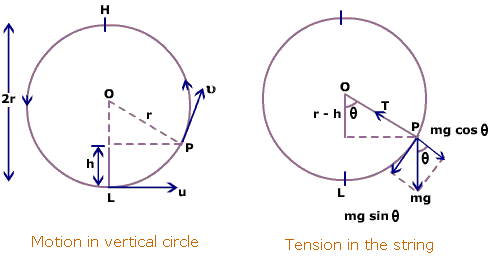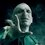# Time Period of Vertical Circular motionNote: $x$ has been used for the angle with the vertical, measured in anti-clockwise direction.

As shown in the figure, the tangential acceleration ${ a }_{ t }$ is $g\sin x$ . Thus, the angular acceleration will be $\frac { { a }_{ t } }{ R }$ , where $R$ is the radius of the circle. Writing $\frac { \omega d\omega }{ dx} =-\dfrac { g\sin x }{ R } int$ $\int _{ v/R }^{ \omega }{ \omega \, d\omega } =\dfrac { g\int _{ 0 }^{ x }{ \, sinx\, dx } }{ R } \\ { This\quad gives\\ \omega =\sqrt { \dfrac { 2g(1-\cos x) }{ R } +{ \dfrac { v }{ R } }^{ 2 } } =\dfrac { dx }{ dt } }$

Now, I don't know how to integrate this expression between $0\quad to\quad 2\pi$, to calculate the time taken for complete oscillation.

Thanks.Note by Abhijeet Verma
5 years, 4 months ago

This discussion board is a place to discuss our Daily Challenges and the math and science related to those challenges. Explanations are more than just a solution — they should explain the steps and thinking strategies that you used to obtain the solution. Comments should further the discussion of math and science.

When posting on Brilliant:

• Use the emojis to react to an explanation, whether you're congratulating a job well done , or just really confused .
• Ask specific questions about the challenge or the steps in somebody's explanation. Well-posed questions can add a lot to the discussion, but posting "I don't understand!" doesn't help anyone.
• Try to contribute something new to the discussion, whether it is an extension, generalization or other idea related to the challenge.

MarkdownAppears as
*italics* or _italics_ italics
**bold** or __bold__ bold
- bulleted- list
• bulleted
• list
1. numbered2. list
1. numbered
2. list
Note: you must add a full line of space before and after lists for them to show up correctly
paragraph 1paragraph 2

paragraph 1

paragraph 2

[example link](https://brilliant.org)example link
> This is a quote
This is a quote
    # I indented these lines
# 4 spaces, and now they show
# up as a code block.

print "hello world"
# I indented these lines
# 4 spaces, and now they show
# up as a code block.

print "hello world"
MathAppears as
Remember to wrap math in $$ ... $$ or $ ... $ to ensure proper formatting.
2 \times 3 $2 \times 3$
2^{34} $2^{34}$
a_{i-1} $a_{i-1}$
\frac{2}{3} $\frac{2}{3}$
\sqrt{2} $\sqrt{2}$
\sum_{i=1}^3 $\sum_{i=1}^3$
\sin \theta $\sin \theta$
\boxed{123} $\boxed{123}$

Sort by:

There is no simple method to evaluate that integral.
Your equation is simply $\dfrac{d^2 \theta}{d t^2} + \dfrac{g \theta}{R} = 0$
The solution of this requires an elliptic integral to be solved.

- 5 years, 4 months ago